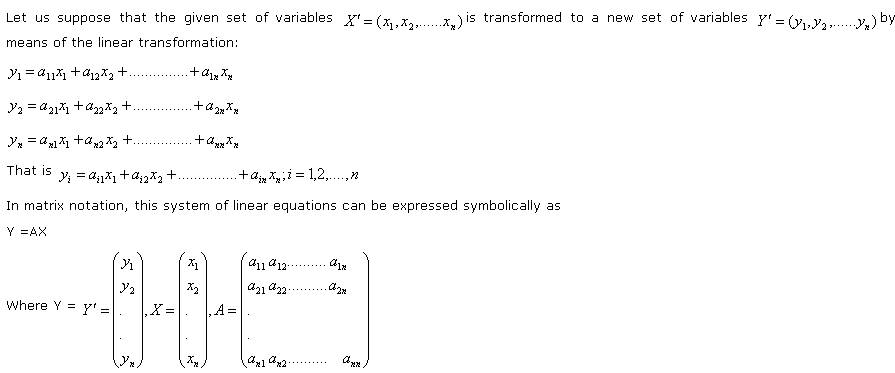# Statistics Assignment Help With Linear Transformation

## 11.6 Linear Transformation:

###From matrix theory, we know that the system has a unique solution if |A| ≠ 0. In other words, we can express X uniquely in terms Y if A is non singular and the solution is given by

X= A-1 Y

Where A-1 is the inverse of the square matrix A.

The linear transformation defined above is said to orthogonal if